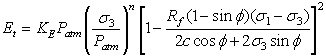Expert Modelling: Hands-on help from Rocscience Engineers. Register Here

# Elastic Properties

The following elastic models are available for defining your material elastic properties in the Define Material Properties dialog:

• Isotropic
• Transversely Isotropic
• Orthotropic
• Duncan-Chang Hyperbolic
• Non-linear Isotropic

## Isotropic

An Isotropic material implies that the material properties do not vary with direction. The elastic properties of an Isotropic material are defined by a single value of Young’s Modulus and a single value of Poisson’s Ratio.

Residual Young's Modulus

For an Isotropic material you can define a residual value of Young's Modulus by selecting the Young's Modulus (residual) check box. This option is only enabled if the Material Type = Plastic and the failure criterion = Mohr-Coulomb, Hoek-Brown or Drucker-Prager. The residual value of Young's Modulus will be used in the analysis under the following conditions:

• Material yielding has occurred (peak strength envelope exceeded)

Only under these conditions will the residual modulus be applied. If the material yields but the load state does not change, then the residual modulus will not be applied. This is indicated in the following figure.

Definition of residual Young's modulusNote: RS2 assumes an abrupt drop from peak to residual strength after failure (i.e. strain softening is not considered). Therefore the residual modulus is NOT the slope of the strain softening curve, since strain softening is not considered.

## Transversely Isotropic

A Transversely Isotropic material has properties which vary with direction. The elastic properties are specified in two orthogonal directions as follows:

• E1 and E2 are the in-plane elastic moduli.
• Ez (the out-of-plane elastic modulus) is assumed to be equal to E1.
• The required Poisson’s ratios are n12 and n1z = nz1 = n. Note that nij implies strain in the j-direction due to strain in the i-direction. Also note that Ei / Ej = nij / nji. Therefore, the plane 1-Z is the isotropic plane
• G12 is the in-plane shear modulus
• The Angle is measured between the x-direction and the direction of E1, as shown in the figure below.

Definition of Angle for Transversely Isotropic or Orthotropic materialsNOTE: you cannot use the Transversely Isotropic elastic model in conjunction with a plasticity analysis in RS2. If you use the Transversely Isotropic elastic model, then only an elastic analysis can be carried out (i.e. you cannot use Material Type = Plastic in Strength Parameters, in conjunction with Elastic Type = Transversely Isotropic).

## Orthotropic

An Orthotropic material has properties which vary with direction. The elastic properties are specified in THREE orthogonal directions.

For an Orthotropic material three Young's moduli are required - E1, E2 and Ez - where the out-of-plane elastic modulus Ez is no longer equal to E1. Three separate Poisson’s ratios are also required, n12, n1z and n2z. The in-plane shear modulus G12 and the Angle definition are the same as for a Transversely Isotropic material.

## Duncan-Chang Hyperbolic

The Duncan-Chang Hyperbolic constitutive model [ Duncan and Chang, (1970) ] is widely used for the modeling of soil behaviour, and is capable of modeling the non-linear, stress-dependent and inelastic behaviour of cohesive and cohesionless soils.

The Duncan-Chang Hyperbolic model can only be used in conjunction with the Mohr-Coulomb failure criterion in RS2.

The following input parameters are used to define the Duncan-Chang Hyperbolic model in RS2:

Modulus Number (Ke) - this dimensionless parameter represents Young's modulus (range of values: 350-1120)

Modulus Exponent (n) - governs the stress dependence of Ke on sigma3 (range of values: 0-1)

Failure Ratio (Rf) - defines the shape of the stress-strain curve (range of values: 0.60-0.95)

Atmospheric Pressure (Patm) - used for normalization of stress input

Poisson's Ratio (v) - can be specified as either Constant or Stress Dependent.

• If Poisson's Ratio = Constant, then Poisson's Ratio is entered directly as a constant value.
• If Poisson's Ratio = Stress Dependent, then the Bulk Modulus parameters are entered (see below).

Bulk Modulus Number (Kb) - this dimensionless parameter characterizes the volumetric change (range of values: 200-700)

Bulk Modulus Exponent (m) - governs the stress dependence of Kb on sigma3 (range of values: 0-1)

Based on a hyperbolic stress-strain curve and stress-dependent material properties, the following equations are derived [ Duncan and Chang, (1970) ] for the Duncan-Chang Hyperbolic model.

The tangential modulus (Et) for a given stress condition, is given by Eqn.1.Eqn.1

The tangential bulk modulus (Bt) and tangential Poisson's ratio are given by Eqn.2 and Eqn.3 respectively.Eqn.2Eqn.3

### Non-linear Isotropic

Non-linear isotropic materials can now be modeled using one of three different non-linear elastic models, see the Theory section for details.